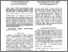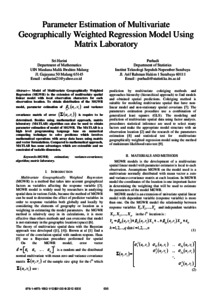# Parameter Estimation of Multivariate Geographically Weighted Regression Model Using Matrix Laboratory

Harini, Sri (2012) Parameter Estimation of Multivariate Geographically Weighted Regression Model Using Matrix Laboratory. Presented at International Conference on Statistics in Science, Business and Engineering (ICSSBE) 2012, 10-12 September 2012, Langkawi, Malaysia.Preview
Text
Paper_ICSSBE2012_108.pdf

Full text available at: https://doi.org/10.1109/ICSSBE.2012.6396622

## Abstract

Model of Multivariate Geographically Weighted
Regression (MGWR) is the extension of multivariate spatial liniear model with local observation characters for each observation location. To obtain distribution of the MGWR model, parameter estimation of B(u,v) and variance covariance matrix of error is require to be determined. Besides using mathematical approach, matrix
laboratory (MATLAB) algorithm can also be used to obtain
parameter estimation of model of MGWR. The MATLAB is a
high level programming language base on numerical
computing technique to solve problems which involves
mathematical operations with array data bases using matrix and vector formulations. Compared to mathematical approach,MATLAB has some advantages which are extensible and no constraint of variable dimension.

Item Type: Conference (Paper) MGWR; estimation; variance-covariance; algorithm; matrix laboratory 01 MATHEMATICAL SCIENCES > 0104 Statistics > 010401 Applied Statistics08 INFORMATION AND COMPUTING SCIENCES > 0802 Computation Theory and Mathematics > 080204 Mathematical Software Faculty of Mathematics and Sciences > Department of Mathematics Dr Sri Harini 27 Jun 2016 19:19View Item